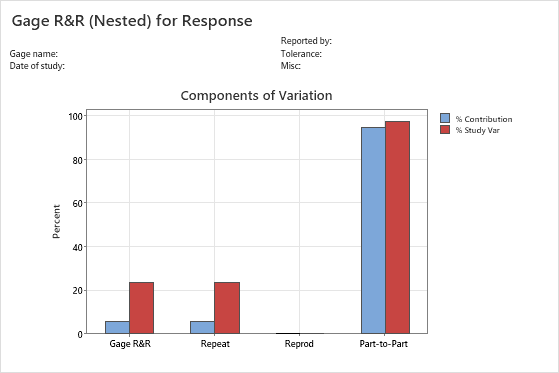# Interpret the key results for Nested Gage R&R Study

Complete the following steps to interpret a nested gage R&R study. Key output includes variability estimates, and graphs of the measurements and measurement variability.

## Step 1: Use the ANOVA table to identify significant factors and interactions

Use the ANOVA table to identify which sources of variability are significant. The ANOVA table includes the following terms in the Source column:
• Operator: The variation that is from the operators.
• Part (Operator): The variation that is from the parts that are nested within each operator.
• Error or repeatability: The variation that is not explained by part or operator.

## Step 2: Assess the variation for each source of measurement error

Use the variance components (VarComp) and %Contribution to assess the variation for each source of measurement error. The sources are as follows:
• Total Gage R&R: The sum of the repeatability and the reproducibility variance components.
• Repeatability: The variability in measurements when the same operator measures parts from the same batch.
• Reproducibility: The variability in measurements when different operators measure the parts.
• Part-to-Part: The variability in measurements due to different parts.
###### Note

If an operator can measure a part only once (such as with destructive testing), you must be able to assume that all parts within a single batch are identical enough to claim that they are the same part. If you are unable to make that assumption then part-to-part variation within a batch will mask the measurement system variation.

Ideally, very little of the variability should be due to repeatability and reproducibility. Differences between parts (Part-to-Part) should account for most of the variability.Key Results: Components of Variation graph The components of variation graph shows the variation from the sources of measurement error. Minitab displays bars for %Tolerance when you enter a tolerance value, and Minitab displays bars for %Process when you enter a historical standard deviation. This graphs shows that most of the variability is from Part-to-Part variation, which indicates that most of the measurement system variation is due to differences between parts.

## Step 3: Examine the graphs for more information on the gage study

The gage R&R graphs provide information about the measurement system.
Components of variation graph
Shows whether the largest of component of variation is part-to-part variation.
In an acceptable measurement system, the largest component of variation is part-to-part variation.
R chart by operator
Shows whether any points fall above the upper control limit.
If the operators measure consistently, the points will fall within the control limits.
Xbar chart by operator
Shows whether most points fall beyond the control limits.
The parts that you choose for a gage R&R study should represent the typical part-to-part variability. Thus, you should expect more variation between part averages, and the graph should show that most points fall beyond the control limits.
Measurements by part (operator) graph
Shows whether multiple measurements for each part by each operator are close together, which indicates between-part variability and within-part variability.
Multiple measurements for each part that are close together indicate small variation between the measurements of the same part measured by the same operator.
Measurements by operator graph
Shows whether differences between operators are small compared to the differences between parts.
A straight horizontal line across operators indicates that the mean measurements for each operator are similar. Ideally, the measurements for each operator vary an equal amount.
By using this site you agree to the use of cookies for analytics and personalized content.  Read our policy